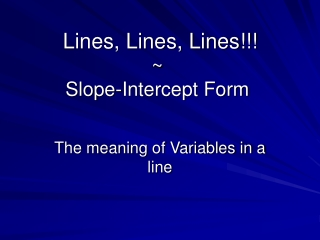Download PresentationLines, Lines, Lines!!! ~ Slope-Intercept Form

# Lines, Lines, Lines!!! ~ Slope-Intercept Form

Télécharger la présentation## Lines, Lines, Lines!!! ~ Slope-Intercept Form

- - - - - - - - - - - - - - - - - - - - - - - - - - - E N D - - - - - - - - - - - - - - - - - - - - - - - - - - -
##### Presentation Transcript

1. Lines, Lines, Lines!!!~Slope-Intercept Form The meaning of Variables in a line

2. Introduction • A Line is composed of an infinite number of points puts together. • All points fit the pattern of a single formula. • There are several ways we could write the formula: • Standard Form • Point-Slope Form • Slope-intercept Form

3. Slope intercept form is: y = mx + b Our goal is to define each line where the “y” is alone on one side of the equation.

4. These equations are all in Slope-Intercept Form: Notice that these equations are all solved for y.

5. y-Intercept slope Now look at the equation below…… When an equation is in slope-intercept form: y = mx + b 2 What is the slope? ____________ 1 What is the intercept? ____________

6. Now Try this Convert to Slope-Intercept Form 2y + 26 = -6x Step 1: Subtract both sides by 26 Step 2: Divide both sides by 2 to get y by itself Step 3: Simplify all fractions - 3 What is the slope? ____________ -13 What is the intercept? ____________

7. y-Intercept slope When an equation is in slope-intercept form: y = mx + b b = y-intercept. This constant helps us identified where to begin our graph. We start our graph on this number of the y-axis. The y-intercept tells us that the line crosses the y-axis at this point. m = slope. This constant is always the coefficient of the ‘x’ variable. It helps us find all of the remaining points of the line

8. On the next three slides we will graph the three equations: using their y-intercepts and slopes.

9. right 1 up 2 right 1 up 2 1. Plot the y-intercept as a point on the y-axis. The constant b = 1, so the y-intercept = 1. 2. Plot more points by counting the slope up the numerator and to the right denominator. The coefficient m = 2, so the slope = 2/1.

10. down 1 down 1 right 1 right 1 1. Plot the y-intercept as a point on the y-axis. The constant b = -4, so the y-intercept = -4. 2. Plot more points by counting the slope up the numerator and right the denominator. The coefficient m = -1, so the slope = -1/1.

11. right 3 up 5 right 3 up 5 1. Plot the y-intercept as a point on the y-axis. The constant b = -7, so the y-intercept = -7. 2. Plot more points by counting the slope up the numerator and to the right denominator. The coefficient m = 5/3, so the slope = 5/3.

12. Graph the following equations: They look different but they are not different. Before we can graph them, it is always best to set them us in the Slope-intercept Form: y = mx + b

13. The constant b = 8 is the y-intercept. The coefficient m = 3/2 is the slope.

14. Or The constant b = 1 is the y-intercept. The coefficient m = 9/7 is the slope.

15. The constant b = -3 is the y-intercept. The coefficient m = -6/5 is the slope.

16. The new modified equations are: Now you will have a much easier time graphing these equations… Now your turn to graph…

17. Student Activity You will now receive a worksheet. Turn the worksheet in when completed.

18. Do Not Disturb Work In Progress